Learn your way!

## Excel 2013 Advanced Stats, Part 4: Regression

#### Watch trailer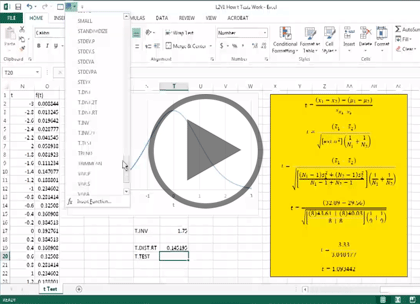#### Course at a glance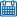Release date 3/18/2015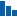Level Advanced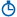Runtime 1h 51m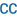Closed captioning N/A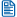Transcript N/A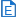eBooks / courseware Included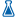Hands-on labs N/A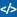Sample code Included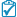Exams N/A##### Enterprise Solutions

Need reporting, custom learning tracks, or SCORM? Learn More

#### Course description

Master statistical analysis with Excel 2013 with this four part learning series of courses . Big data is big business, and many professionals are turning to Excels data analysis tools to help them make sense of their organizational data. Now, you can learn the ins and outs of statistical analysis with Excel – from importing data to utilizing Pivot Tables – with Statistical Analysis with Excel 2013 Advanced Skills. Understand Excels data analysis capabilities and how they can be applied in your organization. Work with data, normal distributions, hypothesis testing, variances, rank, and percentiles. Take advantage of formulas, Pivot Tables, dashboards, and reporting tools to display your data.

#### Prerequisites

This course assumes some familiarity with Microsoft Excel. This course is part of 4 courses; Excel 2013 Advanced Stats: Sample for Mean; Excel 2013 Advanced Stats: F-Test and ANOVA; Excel 2013 Advanced Stats: Trend and Two-Factor; and Excel 2013 Advanced Stats: Regression. Please be sure to view the courses in order if you are new to the material.

#### Meet the expertOur Creative Design courses are presented by experts from Wiley Publishing. Wiley is a global provider of knowledge and knowledge-enabled services that improve outcomes in areas of research, professional practice, and education. They are the publisher of award-winning journals, encyclopedias, books, and online products and services.

#### Regression

##### Introducing Regression (31:21)
• Introduction (00:10)
• Scatterplots (05:03)
• The Regression Line (04:38)
• Regression Line in Excel (04:50)
• Predicted & Residual Values (04:20)
• Sum of Squares & Mean Squares (04:10)
• Test of the Hypothesis (04:11)
• R Squared & R Values (03:44)
• Summary (00:10)
##### Regression Functions (27:43)
• Introduction (00:10)
• Array Names (04:55)
• Calculating with Array Names (04:10)
• Create ANOVA Table (03:31)
• Using the LINEST Function (04:26)
• Regression Data Analysis Tools (03:31)
• Regression Results (02:39)
• T-Test & More Results (04:07)
• Summary (00:10)

#### Regression and Correlation

##### Multiple Regression (22:00)
• Introduction (00:10)
• Multiple Regression (02:37)
• 3D Bubble Plot (03:31)
• Calculate with Trend (04:13)
• LINEST Function (04:02)
• Analysis & Multiple Regression (04:36)
• ANOVA & Residual Output (02:40)
• Summary (00:10)
##### Correlation (15:18)
• Introduction (00:10)
• Correlation Concepts (05:01)
• Covariance of X and Y (02:28)
• Calculate Covariance (03:07)
• Correlation Coefficient (04:20)
• Summary (00:10)
##### Data Analysis for Correlation (14:49)
• Introduction (00:10)
• Apply the Data Analysis Tool (03:48)
• Multiple Correlation (03:13)
• Importing Data from the Web (03:17)
• Applying the Correlation Tool (04:10)
• Summary (00:10)